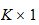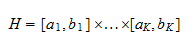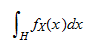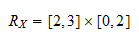StatLect

# Continuous random vector

The concept of continuous random vector is a multivariate generalization of the concept of continuous random variable.

A random vector has the following characteristics:

• the set of values it can take is not countable;

• the probability that its realization will belong to a given set can be computed as a multiple integral over that set of a function called joint probability density function.## Synonyms

A continuous random vector is sometimes also called absolutely continuous.

## Definition

The following is a formal definition.

Definition Arandom vectoris said to be continuous if the set of values it can take is not countable and the probability thattakes a value in a given hyper-rectanglecan be expressed as a multiple integral:where the integrand functionis called the joint probability density function of.

With vector notation, the multiple integral above could also be written in more compact form aswhich makes clear that the joint probability density function is a straightforward multivariate generalization of the univariate probability density function.

A continuous random vector is often said to have a multivariate continuous distribution.

## Example

Letbe acontinuous random vector that can take values in the setLet its joint probability density function beSuppose we need to compute the probabilityThis can be calculated as a multiple integral:## Common multivariate continuous distributions

The multivariate normal distribution and the multivariate Student distribution are examples of multivariate continuous distributions.

## More details

More details about continuous random vectors can be found in the lecture entitled Random vectors.

Previous entry: Absolutely continuous random variable